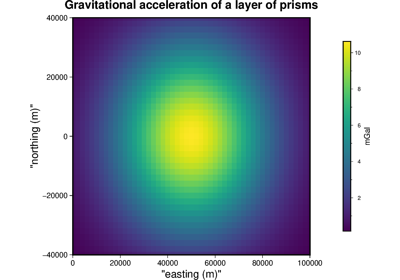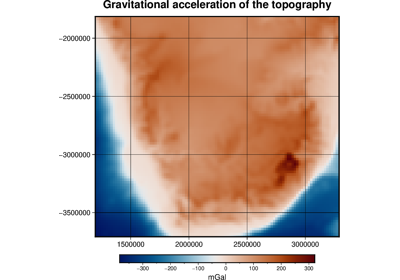# harmonica.prism_gravity¶

harmonica.prism_gravity(coordinates, prisms, density, field, parallel=True, dtype='float64', progressbar=False, disable_checks=False)[source]

Gravitational fields of right-rectangular prisms in Cartesian coordinates

The gravitational fields are computed through the analytical solutions given by [Nagy2000] and [Nagy2002], which are valid on the entire domain. This means that the computation can be done at any point, either outside or inside the prism.

This implementation makes use of the modified arctangent function proposed by [Fukushima2020] (eq. 12) so that the potential field to satisfies Poisson’s equation in the entire domain. Moreover, the logarithm function was also modified in order to solve the singularities that the analytical solution has on some points (see [Nagy2000]).

Warning

The z direction points upwards, i.e. positive and negative values of `upward` represent points above and below the surface, respectively. But remember that the `g_z` field returns the downward component of the gravitational acceleration so that positive density contrasts produce positive anomalies.

Parameters
• coordinates (list or 1d-array) – List or array containing `easting`, `northing` and `upward` of the computation points defined on a Cartesian coordinate system. All coordinates should be in meters.

• prisms (list, 1d-array, or 2d-array) – List or array containing the coordinates of the prism(s) in the following order: west, east, south, north, bottom, top in a Cartesian coordinate system. All coordinates should be in meters. Coordinates for more than one prism can be provided. In this case, prisms should be a list of lists or 2d-array (with one prism per line).

• density (list or array) – List or array containing the density of each prism in kg/m^3.

• field (str) –

Gravitational field that wants to be computed. The available fields are:

• Gravitational potential: `potential`

• Downward acceleration: `g_z`

• parallel (bool (optional)) – If True the computations will run in parallel using Numba built-in parallelization. If False, the forward model will run on a single core. Might be useful to disable parallelization if the forward model is run by an already parallelized workflow. Default to True.

• dtype (data-type (optional)) – Data type assigned to the resulting gravitational field. Default to `np.float64`.

• progressbar (bool (optional)) – If True, a progress bar of the computation will be printed to standard error (stderr). Requires `numba_progress` to be installed. Default to `False`.

• disable_checks (bool (optional)) – Flag that controls whether to perform a sanity check on the model. Should be set to `True` only when it is certain that the input model is valid and it does not need to be checked. Default to `False`.

Returns

result (array) – Gravitational field generated by the prisms on the computation points.

Examples

Compute gravitational effect of a single a prism

```>>> # Define prisms boundaries, it must be beneath the surface
>>> prism = [-34, 5, -18, 14, -345, -146]
>>> # Set prism density to 2670 kg/m³
>>> density = 2670
>>> # Define three computation points along the easting axe at 30m above
>>> # the surface
>>> coordinates = ([-40, 0, 40], [0, 0, 0], [30, 30, 30])
>>> # Compute the downward component of the gravitational acceleration that
>>> # the prism generates on the computation points
>>> gz = prism_gravity(coordinates, prism, density, field="g_z")
>>> print("({:.5f}, {:.5f}, {:.5f})".format(*gz))
(0.06551, 0.06628, 0.06173)
```

Define two prisms with positive and negative density contrasts

```>>> prisms = [[-134, -5, -45, 45, -200, -50], [5, 134, -45, 45, -180, -30]]
>>> densities = [-300, 300]
>>> # Compute the g_z that the prisms generate on the computation points
>>> gz = prism_gravity(coordinates, prisms, densities, field="g_z")
>>> print("({:.5f}, {:.5f}, {:.5f})".format(*gz))
(-0.05379, 0.02908, 0.11235)
```

## Examples using `harmonica.prism_gravity`¶